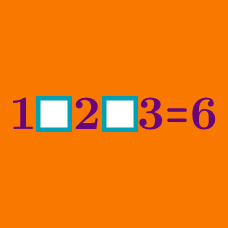Logic

# Operator Search: Level 2 Challenges

$\large 1 \, \square \, 2 \, \square \, 3 \, \square \, 4 \, \square \, 5 \, \square \, 6 \, \square \, 7 \, \square \, 8 = 9$

There are $$2^7 =128$$ ways in which we can fill the squares with $$+, -$$.

How many ways would make the equation true?

Note: You are not allowed to use parenthesis.

$\large 3 \; \square \; 3 \; \square \; 3 \; \square \; 3$

Fill in the boxes above with any of the four mathematical operators ( $$+, -, \times , \div$$ ). Which of the following cannot be a resultant number?

Note: Order of operations (BODMAS) applied.

$\large 1 \; \square \; 2 \; \square \; 3\; \square \; 4\; \square \; 5 \; \square \; 6 \; \square \; 7 \; \square \; 8 \; \square \; 9 = 33$

Seven of the eight "$$\square$$"s above are filled with "$$+$$", and the other one with "$$-$$".

Before which integer should the "$$-$$" sign be placed to make the equation true?

$\large 18 \ \square \ 12 \ \square \ 4 \ \square \ 5 = 59$

Replace each $$\square$$ with one of $$+,-,\times,\div$$ to make the equation true.

Submit your answer as a 3 digit number, where:

• $$1$$ represents $$+$$
• $$2$$ represents $$-$$
• $$3$$ represents $$\times$$
• $$4$$ represents $$\div$$

Example : If your answer is $$+, -, \times$$ then enter the answer as $$123$$.

$\Huge 1\ \ \ \ 4 \ \ \ \ 9 \ \ = \ \ 16$

Is it possible to make this equation true by inserting the appropriate operations? Any operations and functions can be used.

×

Problem Loading...

Note Loading...

Set Loading...